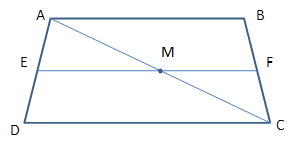Chapter 4.4, Problem 33E### Elementary Geometry for College St...

6th Edition
Daniel C. Alexander + 1 other
ISBN: 9781285195698

#### Solutions

Chapter
Section### Elementary Geometry for College St...

6th Edition
Daniel C. Alexander + 1 other
ISBN: 9781285195698
Textbook Problem
30 views

# In exercises 27 to 33, complete a formal proof.Given: E F ¯ is the median of trapezoid ABCD.Prove: E F = 1 2 ( A B + D C ) .(HINT: Using theorem 4.4.7, show that M is the midpoint of A C ¯ . For Δ A D C         a n d           Δ C B A apply theorem 4.2.5)To determine

To Prove:

EF=12(AB+DC)

Explanation

Consider the following diagram.

Given: EF¯ is the median of the trapezoid ABCD.

Prove: EF=12(AB+DC).

Plan: Draw a transversal AC¯ through M.

Here, AB||EF||DC.

We know that, three parallel lines intercept congruent segments on one transversal, then they intercept congruent segments on any transversal.

It means AMMC.

Therefore, M is the midpoint of AC¯

### Still sussing out bartleby?

Check out a sample textbook solution.

See a sample solution

#### The Solution to Your Study Problems

Bartleby provides explanations to thousands of textbook problems written by our experts, many with advanced degrees!

Get Started

#### (x3y2z2)2

Applied Calculus for the Managerial, Life, and Social Sciences: A Brief Approach

#### In Exercises 116, determine whether the argument is valid. pqqp

Finite Mathematics for the Managerial, Life, and Social Sciences

#### log27 9 =

Study Guide for Stewart's Single Variable Calculus: Early Transcendentals, 8th

#### True or False: is a convergent series.

Study Guide for Stewart's Multivariable Calculus, 8th